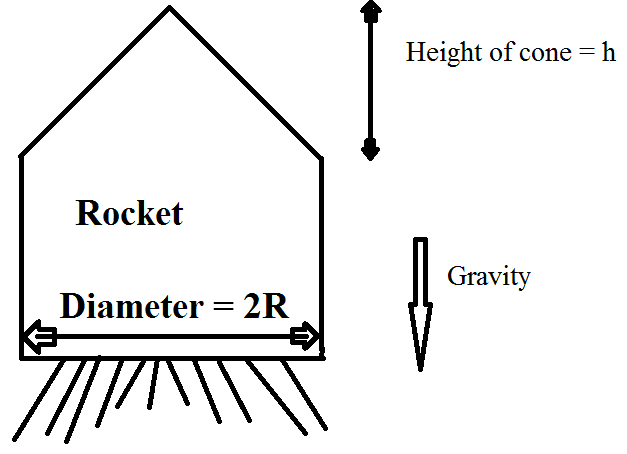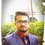# Need help In Rocket Physics (1)Image

Fuel is ejected from a rocket of mass ${ M }_{ 0 }$ at a velocity $u$ $m/s$ relative to Rocket. Fuel is ejecting at constant rate of $\mu \quad Kg/s$. Initial Velocity rocket starts from rest from the surface of earth

( Neglect air and other resistance force in both Cases)

Q1). Assume gravity is constant , Find acceleration, velocity and Thrust as a function of time.

Q2). Assume gravity changes with height $h$ from earth surface as $g\left( h \right) =\frac { g }{ { \left( 1+\frac { h }{ { R }_{ e } } \right) }^{ 2 } }$, Find acceleration, Velocity and Thrust as a function of time.Note by Rishabh Deep Singh
3 years, 10 months ago

This discussion board is a place to discuss our Daily Challenges and the math and science related to those challenges. Explanations are more than just a solution — they should explain the steps and thinking strategies that you used to obtain the solution. Comments should further the discussion of math and science.

When posting on Brilliant:

• Use the emojis to react to an explanation, whether you're congratulating a job well done , or just really confused .
• Ask specific questions about the challenge or the steps in somebody's explanation. Well-posed questions can add a lot to the discussion, but posting "I don't understand!" doesn't help anyone.
• Try to contribute something new to the discussion, whether it is an extension, generalization or other idea related to the challenge.

MarkdownAppears as
*italics* or _italics_ italics
**bold** or __bold__ bold
- bulleted- list
• bulleted
• list
1. numbered2. list
1. numbered
2. list
Note: you must add a full line of space before and after lists for them to show up correctly
paragraph 1paragraph 2

paragraph 1

paragraph 2

[example link](https://brilliant.org)example link
> This is a quote
This is a quote
    # I indented these lines
# 4 spaces, and now they show
# up as a code block.

print "hello world"
# I indented these lines
# 4 spaces, and now they show
# up as a code block.

print "hello world"
MathAppears as
Remember to wrap math in $$ ... $$ or $ ... $ to ensure proper formatting.
2 \times 3 $2 \times 3$
2^{34} $2^{34}$
a_{i-1} $a_{i-1}$
\frac{2}{3} $\frac{2}{3}$
\sqrt{2} $\sqrt{2}$
\sum_{i=1}^3 $\sum_{i=1}^3$
\sin \theta $\sin \theta$
\boxed{123} $\boxed{123}$

Sort by:

Use impulse momentum theoerem in both cases Consider any arbitrary moment in time t and distance y from surface of earth Let us consider that rocket as my system with mass at that point in spacetime as m In time dt let dm mass be ejected absolute velocity of dm is u-v where u is the relative velocity and v is the absolute velocity of rocket at that moment Impulse due to weight is mgdt which is the change in momentum of the system that is rocket After this we form a differentia equation which is dificult to solve in case 2 I am not able to solve the equation In case 1 V= uln(m■\m) -g\p(m■-m) Where m■ is initia mass p is dm\dt

- 2 years, 8 months ago

Is acceleration $\frac{\mu u}{M_{0} -\mu t}$ ?

- 3 years, 10 months ago

How do you find it ?? I don't know the answer.

- 3 years, 10 months ago

Use conservation of momentum and a=dv/dt.

- 3 years, 10 months ago

You are totally wrong.

We can't apply conservation of momentum as there is gravity and also mass is changing with Time.

- 3 years, 10 months ago